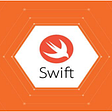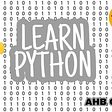# The Law of Cosines

## If you are trying to understand trigonometry you should definitely read this. This theorem is a commonly used trick for some problems that require pretty genius and creative geometric problems.

In this article, I will be proving the law of cosines. If you know a little about triangles and angles you can do it yourself! So, before reading the proof, you had better try to prove it.

## The Law of Cosines

Let’s start with the theorem. In any type of triangle, the following equation holds.

## What does cosα mean?

As you can understand from the name of the theorem it has something to do with cosines. But what is that “cosα”? Cosines is a trigonometric function that is equal to the ratio of the side adjacent to an acute angle (in a right-angled triangle) to the hypotenuse.

## Proof

Now, the proof is coming. Make sure you have dealt with the problem before you read the proof.

Let h be the length of the height of the triangle from vertex B. Let y be the length of the right side of the height. By definition, cosα = y/c. Then we can write a² = h² + (b-y)² from Pythagoras theorem. Also, we know c² = h² + y² from Pythagoras theorem. If we write c² -y² instead of h, we will get a² = c² + b² -2by. We want to use cosα somewhere to get c² + b² -2bc(cosα). If we write -2bc.y/c instead of -2by, we will get -2bc(cosα). This gives us the equality which we were trying to prove.

Same proof occurs if there is an obtuse triangle. I need to note that,

cosα=-cos(180-α)

and this comes from the unit circle.

Resources,

1 The Law of Cosines. WolframMathWorld. Web. 28.04.2020 (Link)

--

--

--

## More from Betamat - EN

Betemat is a creation that aims to produce maths-related content.

## My experiences with Unity and Unreal## Top 10 swift projects on github## Lawsuits and Trials## Special offers for Launching the first application of DeFi.ECO## Certified Kubernetes Administrator (CKA) 2020 Exam Changes## 6 Tech Tricks You Need in Your Back Pocket## Let’s talk let’s connect | LTLC0166 | July 28, 2020 | Python -Learn from fundamental | AHB-Coders## Ceren Şahin

Co-founder at Betamat, YGA volunteer, table tennis passionate, web developer and a curious coder.

## More from Medium## Emmy Noether, alphabetic portrait## “Developing Me”